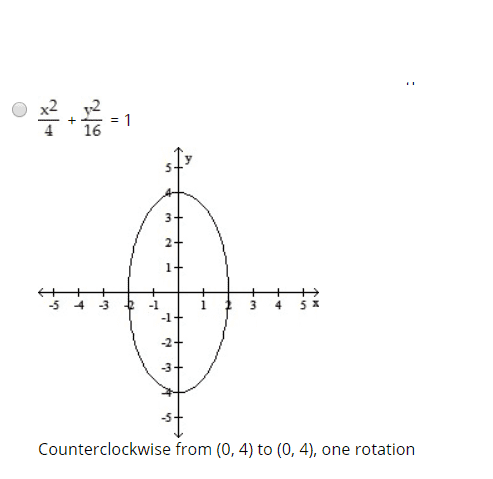# Graph the Cartesian equation: x = 2 sin t, y = 4 cos t

## Homework Statement

Parametric equations and a parameter interval for the motion of a particle in the xy-plane are given. Identify the particle's path by finding a Cartesian equation for it. Graph the Cartesian equation. Indicate the portion of the graph traced by the particle and the direction of motion.

x = 2 sin t, y = 4 cos t, 0 ≤ t ≤ 2π

## Homework Equations

##cos^2t + sin^2t=1##

## The Attempt at a Solution

## x= 2 sin t ##
## y = 4 cos t ##
Square both sides :
## \frac{x^2}{4} = sin t^2 ##
## \frac{y^2}{16} = cos^2 t ##
## \frac{y^2}{16} + \frac{x^2}{4} = 1 ##
This equation forms an ellipse.
When t = 0 , x = 0 and y = 4
When t = ##2\pi## , x = 0 and y = 4
Counterclockwise from (0,4) to (0,4) , one rotation .Could someone check my answer ?

Mark44
Mentor

## Homework Statement

Parametric equations and a parameter interval for the motion of a particle in the xy-plane are given. Identify the particle's path by finding a Cartesian equation for it. Graph the Cartesian equation. Indicate the portion of the graph traced by the particle and the direction of motion.

x = 2 sin t, y = 4 cos t, 0 ≤ t ≤ 2π

## Homework Equations

##cos^2t + sin^2t=1##

## The Attempt at a Solution

## x= 2 sin t ##
## y = 4 cos t ##
Square both sides :
## \frac{x^2}{4} = sin t^2 ##
## \frac{y^2}{16} = cos^2 t ##
•